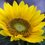Is This A New Formula For Infinitely Nested Radicals?

$x+a=\sqrt{a(x^2+3ax+a^2)+x^2\sqrt{2a(x^2+3(2a)x+(2a)^2)+x^2\sqrt{4a(x^2+3(4a)+(4a)^2)+x^2\dotsm}}}$ $Derivation:$I made use of the following identity; $x+a=\sqrt{(x+a)^3}\\=\sqrt{x^3+3ax^2+3a^2x+a^3}\\=\sqrt{x^3+2ax^2+ax^2+3a^2x+a^3}\\=\sqrt{ax^2+3a^2x+a^3+x^3+2ax^2}\\=\sqrt{a(x^2+3ax+a^2)+x^2(x+2a)}$ In the 2nd step I wrote $3ax^2$ as $2ax^2+ax^2$.In the 3rd step I rearranged the terms.In the 4th step I factored out the $a$ from the first 3 terms and I factored out the $x^2$ from the last 2 terms.Manipulating $x+2a=\sqrt{x^3+3(2a)x^2+3(2a)^2x+(2a)^3}$ in the same way,I got $x+2a=\sqrt{2a(x^2+3(2a)x+(2a)^2)+x^2(x+4a)}$.In the same way I got the expression for $x+4a,x+8a,x+16a\dotsm$.Now I observed that each of these can be substituted in the previous expression.Substituting my way upto $x+a$,I got the expression written at the beginning.Now,I want the people on Brilliant to verify this expression and to see whether it works.Please help me!!!Note by Abdur Rehman Zahid
4 years, 11 months ago

This discussion board is a place to discuss our Daily Challenges and the math and science related to those challenges. Explanations are more than just a solution — they should explain the steps and thinking strategies that you used to obtain the solution. Comments should further the discussion of math and science.

When posting on Brilliant:

• Use the emojis to react to an explanation, whether you're congratulating a job well done , or just really confused .
• Ask specific questions about the challenge or the steps in somebody's explanation. Well-posed questions can add a lot to the discussion, but posting "I don't understand!" doesn't help anyone.
• Try to contribute something new to the discussion, whether it is an extension, generalization or other idea related to the challenge.
• Stay on topic — we're all here to learn more about math and science, not to hear about your favorite get-rich-quick scheme or current world events.

MarkdownAppears as
*italics* or _italics_ italics
**bold** or __bold__ bold

- bulleted
- list

• bulleted
• list

1. numbered
2. list

1. numbered
2. list
Note: you must add a full line of space before and after lists for them to show up correctly
paragraph 1

paragraph 2

paragraph 1

paragraph 2

> This is a quote
This is a quote
# I indented these lines
# 4 spaces, and now they show
# up as a code block.

print "hello world"
# I indented these lines
# 4 spaces, and now they show
# up as a code block.

print "hello world"
MathAppears as
Remember to wrap math in $$...$$ or $...$ to ensure proper formatting.
2 \times 3 $2 \times 3$
2^{34} $2^{34}$
a_{i-1} $a_{i-1}$
\frac{2}{3} $\frac{2}{3}$
\sqrt{2} $\sqrt{2}$
\sum_{i=1}^3 $\sum_{i=1}^3$
\sin \theta $\sin \theta$
\boxed{123} $\boxed{123}$

Sort by:

Ah yes. Isn't it amazing how much can be achieved in a year?

Staff - 3 years, 9 months ago

Yeah.I didn't even know a thing back then.Brilliant has helped me a lot

- 3 years, 9 months ago

if a=0 then x also equal to 0 as x-x=0

- 4 years, 9 months ago

Put a = 0

R.H.S becomes 0 but L.H.S is still 'x'

So I don't think it's correct

- 4 years, 11 months ago

Putting $a \,=\, 0$ yields another infinitely nested series of $x$, which on simplification is equal to $x$.

- 3 years, 6 months ago

Yup

- 3 years, 6 months ago

Because you are dealing with infinite sums/roots, you have to be really careful of what the proper interpretation is.

Of course, it could be that the statement is not true for $a = 0$, but is true for all other real numbers.

Staff - 4 years, 11 months ago

Just looking back at this note and thinking......That was a long time ago

- 3 years, 9 months ago

Please explain how R.H.S becomes zero

- 4 years, 11 months ago

Every term on R.H.S is multiple of 'a' you can see itself

- 4 years, 11 months ago

If we rearrange the formula for $x+a=\sqrt{x^3+3ax^2+3a^2x+a^3}$, you get $x+a=\sqrt{a(x^2+3ax+a^2)+x^2(x+2a)}$ In this expression if you replace a by 2a, you get $x+2a=\sqrt{2a(x^2+3(2a)x+(2a)^2)+x^2(x+4a)}$Replacing this expression in the expression for x +a, you get $x+a=\sqrt{a(x^2+3ax+a^2)+x^2\sqrt{2a(x^2+3(2a)x+(2a)^2)+x^2(x+4a))}}$In this way if we continue deriving the expression for x+4a,x+8a,x+16a etc and replacing them in the original formula for x+a we will get the original formula .So R.H.S does not become zero you just have to replace the value of x+2a instead of the next radical and then set a=0 and after simplifying you get $x=\sqrt{x^3}$

- 4 years, 11 months ago